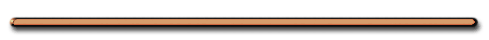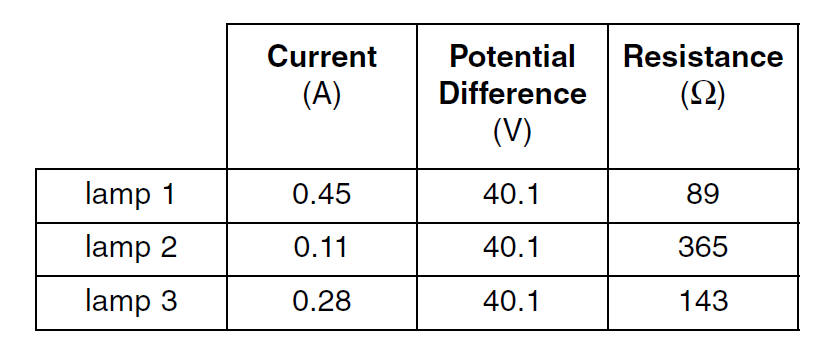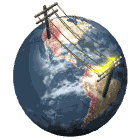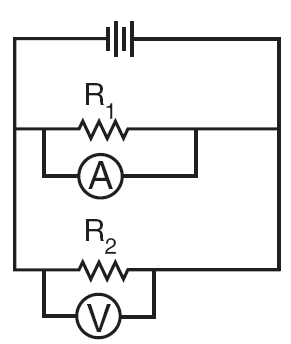Try this at Home!

Series or Parallel?June 2003 Question 63

Answer - Parallel - same VElectric Energy and Heat

(W) Electric energy consumed or released as heat

(All equations on reference table)

(J) W = Pt = [V2/R]t

= VIt = I2Rt

 An electric iron operating at 120 volts draws 10. amperes of current. How much heat energy is delivered by the iron in 30. seconds?a) 1.2 x 103 J c) 3.6 x 104 J b) 3.0 x 102 J d) 3.6 x 103 J

V = 120 V

I = 10. A

t = 30. sec

W = ?

W = VIt

= 120. V(10. A)(30. sec)

W = 3.6 x 104 JWhat's wrong with this picture?Ammeter should be  in series with resistor

Show Me The Physics
Animations

Circuits - Series and Parallel

Electricity Unit Review